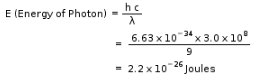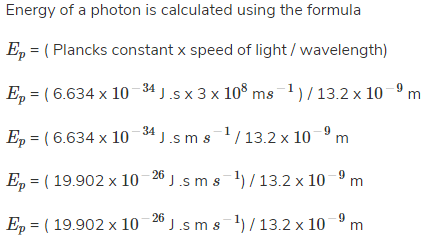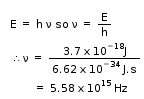NEET  >  Test: Planck’s Quantum Theory

# Test: Planck’s Quantum Theory

Test Description

## 15 Questions MCQ Test Chemistry Class 11 | Test: Planck’s Quantum Theory

Test: Planck’s Quantum Theory for NEET 2023 is part of Chemistry Class 11 preparation. The Test: Planck’s Quantum Theory questions and answers have been prepared according to the NEET exam syllabus.The Test: Planck’s Quantum Theory MCQs are made for NEET 2023 Exam. Find important definitions, questions, notes, meanings, examples, exercises, MCQs and online tests for Test: Planck’s Quantum Theory below.
Solutions of Test: Planck’s Quantum Theory questions in English are available as part of our Chemistry Class 11 for NEET & Test: Planck’s Quantum Theory solutions in Hindi for Chemistry Class 11 course. Download more important topics, notes, lectures and mock test series for NEET Exam by signing up for free. Attempt Test: Planck’s Quantum Theory | 15 questions in 15 minutes | Mock test for NEET preparation | Free important questions MCQ to study Chemistry Class 11 for NEET Exam | Download free PDF with solutions
 1 Crore+ students have signed up on EduRev. Have you?
Test: Planck’s Quantum Theory - Question 1

### The energy of a photon in Joules that has a wavelength of 9.0 m is:

Detailed Solution for Test: Planck’s Quantum Theory - Question 1Test: Planck’s Quantum Theory - Question 2

### The ratio of the energy of a photon of 2000 Çº wavelength radiation to that of 4000 Çº radiation is:

Detailed Solution for Test: Planck’s Quantum Theory - Question 2

We have E = hc/λ
E1/E2 = 4000Å/2000Å = 2

Test: Planck’s Quantum Theory - Question 3

### A radio operator broadcast on the 6-meter band. The frequency of this electromagnetic radiation is __________ MHz.

Detailed Solution for Test: Planck’s Quantum Theory - Question 3

The speed of all form of electromagnetic wave is related by the equation c = λ.ν,

Where c is the speed of light = 3.00 x 108 m/s, λ is the wavelength in m and ν is the frequency is s-1 or Hz.

Therefore, ν = 3.0 x108 / 6 = 5 x 108 s-1 = 50 MHz

Test: Planck’s Quantum Theory - Question 4

Electromagnetic radiation travels through vacuum at a speed of __________ m/s.

Detailed Solution for Test: Planck’s Quantum Theory - Question 4

The speed of light (electromagnetic radiation) through vacuum has a constant value of 3.00 x 108m/s and is independent of the wavelength in vacuum.

Test: Planck’s Quantum Theory - Question 5

What is the frequency of light in s-1 that has a wavelength of 3.12 x 10-3 cm?

Detailed Solution for Test: Planck’s Quantum Theory - Question 5

The wavelength (λ) and frequency (ν) of electromagnetic wave is related by the equation c = λ.ν,

Where c is the speed of light = 3.00 x 108 m/s, λ is the wavelength in m and ν is the frequency is s-1or Hz.

Substituting the values in the formula, we get,

c = λ.ν

ν = c/λ

ν = 3.0 x 108 / 3.12 x 10-5

= 0.96 x 1013 m =  9.62 X 1012

Test: Planck’s Quantum Theory - Question 6

The ejected electrons from the surface of metal in photoelectric effect are called:

Detailed Solution for Test: Planck’s Quantum Theory - Question 6

If light beyond a specific threshold frequency hits a metal surface, electrons are ejected from the surface of the metal. The minimum energy required to eject an electron from the surface is called the work function of the metal. These ejected electrons are called photoelectrons. The kinetic energy of the most energetic electron among these photoelectrons is called the maximum kinetic energy.

Test: Planck’s Quantum Theory - Question 7

If the value of azimuthal quantum number is 3, the possible values of magnetic quantum numbers would be:

Detailed Solution for Test: Planck’s Quantum Theory - Question 7

The total possible values of m for l range from – through 0 to +.

Test: Planck’s Quantum Theory - Question 8

What is the energy of a photon that has a wavelength of 13.2 nm?

Detailed Solution for Test: Planck’s Quantum Theory - Question 8= 1.507 x 10-15J.

Test: Planck’s Quantum Theory - Question 9

What is correct about wave number?

Detailed Solution for Test: Planck’s Quantum Theory - Question 9

Wavenumber is defined as the number of complete wave cycles per unit length of a linear space. It can also be referred to as the spatial frequency of the waves represented in radians per unit distance, or cycles per unit distance. It is usually measured in units of reciprocal centimeters (cm-1) and reciprocal meters (m-1).

Test: Planck’s Quantum Theory - Question 10

The wavelength of light that has a frequency of 1.20 × 1013s-1 is __________ m.

Detailed Solution for Test: Planck’s Quantum Theory - Question 10

The speed of all form of electromagnetic wave is related by the equation c = λ.v, whereby, λ = 3.0 x 108 / 1.2 x 1013 = 2.5 x 10 -5 m.

Test: Planck’s Quantum Theory - Question 11

Which of the following radiation has the shortest wavelength.

Detailed Solution for Test: Planck’s Quantum Theory - Question 11

Radio waves, infrared rays, visible light, ultraviolet rays, X-rays, and gamma raysare all types of electromagnetic radiation. Radio waves have the longest wavelength, and gamma rays have the shortest wavelength.

Test: Planck’s Quantum Theory - Question 12

Who discovered and first used the constant h = 6.6 x 10-34 J.s?

Detailed Solution for Test: Planck’s Quantum Theory - Question 12

Max Planck gave the Planck’s quantum theory and was the first to determine the value of h which is the Planck’s constant.

Test: Planck’s Quantum Theory - Question 13

The energy of a photon of light has what kind of proportionality to its frequency and its wavelength.

Detailed Solution for Test: Planck’s Quantum Theory - Question 13

As E=h(frequency) E is directly proportional to frequency and as frequency = speed of light / wavelength frequency is inversely proportional to wavelength.

Test: Planck’s Quantum Theory - Question 14

What is the wavelength of light (nm) that has a frequency 4.62 ×1014s-1?

Detailed Solution for Test: Planck’s Quantum Theory - Question 14

The wavelength (λ) and frequency (ν) of electromagnetic wave is related by the equation c = λ.ν, whereby, λ = 3.0 x108 / 4.62 ×1014 =  0.649 x 10-6 m = 649nm

Test: Planck’s Quantum Theory - Question 15

What is the frequency of a photon that has an energy of 3.7 x 10-18J?

Detailed Solution for Test: Planck’s Quantum Theory - Question 15## Chemistry Class 11

204 videos|331 docs|229 tests
 Use Code STAYHOME200 and get INR 200 additional OFF Use Coupon Code
Information about Test: Planck’s Quantum Theory Page
In this test you can find the Exam questions for Test: Planck’s Quantum Theory solved & explained in the simplest way possible. Besides giving Questions and answers for Test: Planck’s Quantum Theory, EduRev gives you an ample number of Online tests for practice

## Chemistry Class 11

204 videos|331 docs|229 tests

### How to Prepare for NEET

Read our guide to prepare for NEET which is created by Toppers & the best Teachers